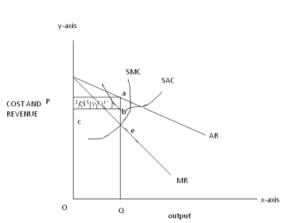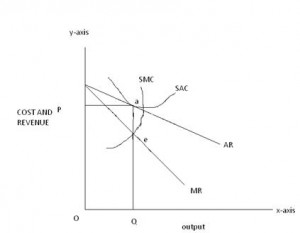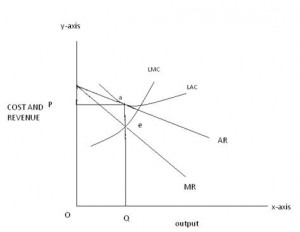# Equilibrium of a Firm Under Monopolistic Competition

EQUILIBRIUM OF A FIRM UNDER MONOPOLISTIC COMPETITION:-

Short run equilibrium: – in the short run, the number of firms remains the same. Each firm wants to earn maximum profit. Under monopolistic in the short run, some firms can fix different prices. Keeping in view their total cost, sometimes, new firms earn abnormal profit than others by advertising their products.

In the monopolistic competition also, a firm is in equilibrium position when

MC= MR

MC cuts MR from below.

The case of above normal profit: – As shown in the following figure, the firm meets the equilibrium condition at point “e”, where it is producing OQ amount of output at price OP. The price at the quantity OQ is found by looking at the average revenue curve (AR) at the point “a”. The profit is shown by the shaded area “cbap”, which is seen by looking at the difference between average cost and average revenue.NORMAL PROFIT: – In the following figure, the firm earns normal profits only. Here average costs are equal to the average revenue, which is shown by point “a”.THE CASE OF LOSS: – when the costs are greater than revenues, the firm is facing loss.  In the following figure the firm is in equilibrium at point “e” producing OQ output at OP price. The average cost at OQ, output is OC, which is greater than average revenue OP. the firm is incurring loss equal to the shaded area “pabc” which is the difference between total cost “oqbc” and total revenue “oqap”LONG RUN ANALYSIS: – entry and exit of firms in a monopolistic competitive industry as under the perfect competition so this will lead to the existence of only normal profits and no firm earn abnormal profits of losses. And each firm produces similar products. Earning of normal profits and shown in the figure below: –#### About rizwan

View all posts by rizwan →